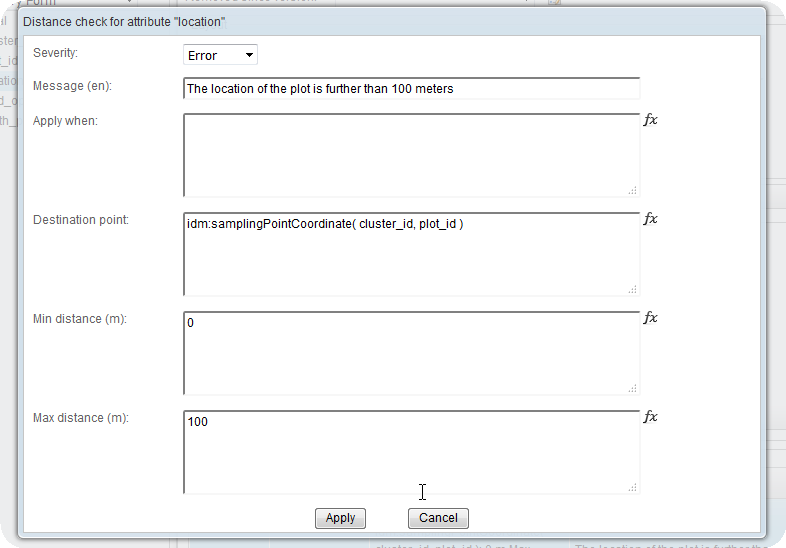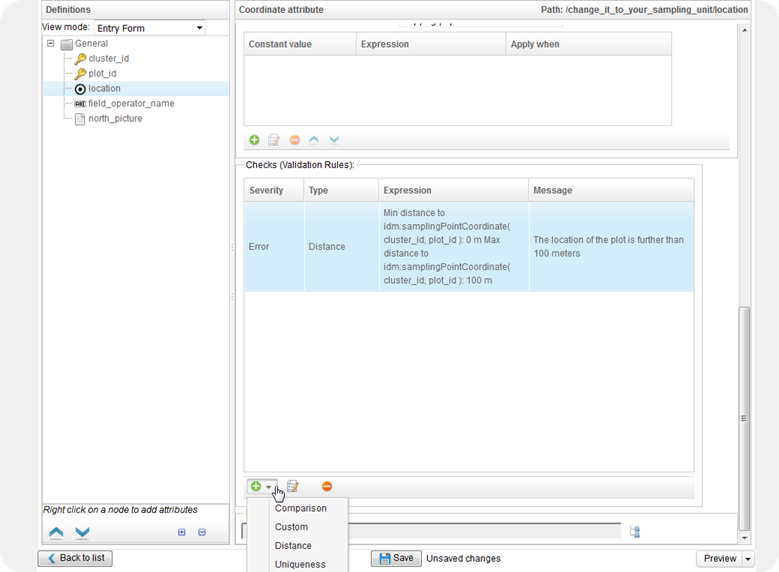# Collect Mobile : how to check distance to plot?

 0 Hi, I would like to show a warning if the operator using Collect Mobile is further than 20 meters from the center of the plot that has been specified in the sampling design. So I have a very simple case, a survey that is using the same sampling design as can be downloaded as an example from the Collect Survey Designer (the one in VietNam) and that has cluster and plot IDs as key fields and then ask the user to take a picture, write his/her name and record his current location. How can I show a warning if the current location is further than 20 meters from the expected location of the center of the plot??? This is the Collect Survey file ( and here the Collect Mobile one in case you want to test) Thank you!! asked 21 Mar '16, 15:56collector 26●6●16●23 accept rate: 0%

 1 Hi, we just did this for a survey in Uganda. For your plot location add into 'Checks (Validation rule)' a warning and the following formula into 'Destination point': idm:samplingPointCoordinate( parent()/parent()/cluster_id, parent()/plot_id ) and then set 'Max distance' to 20 In our case the plot coordinate was under the similar hierachy (cluster/plot). answered 23 Mar '16, 06:52LauriV ♦♦ 590●2●8●13 accept rate: 21%
 0 Thank you very much for your answer LauriV So it seems that my survey is a bit different because the cluster_id and plot_id are in the same entity(not very good design I guess) so instead of using the parent() to access the parent entity I reference the IDs straight away. `idm:samplingPointCoordinate( cluster_id, plot_id )` I guess that normally the Cluster would be an entity and Plot a "child" entity so I would have to refer to the `cluster_id` as `parent()/cluster_id` if my `location` attribute is in the same entity as the `plot_id` ... Anyway here is how I did it in pictures :answered 23 Mar '16, 09:26collector 26●6●16●23 accept rate: 0%
 0 On this topic, and after looking into this survey ( showcase Collect survey ) I found that I can also have a calculated field used to find what is the distance between the plot_center and the location where the operator took the measurements. So first you need to have a calculated coordinate attribute that gets the center plot location using an expression like this: ``````idm:samplingPointCoordinate(cluster_id, plot_id) `````` And then you will need to have another calculated number attribute (type real) that will actually perform the distance operation using an expression such as this one ( where location is the attribute that is used to record the location using the device GPS and sampling_point_location is the calculate coordinate that we just added): ``````idm:distance(sampling_point_location, location) `````` You can find more about these functions here : LINK ABOUT IDM FUNCTIONS Also this is the final result of my test survey! LINK answered 23 Mar '16, 10:01collector 26●6●16●23 accept rate: 0%
 toggle preview

By Email:

Markdown Basics

• *italic* or _italic_
• **bold** or __bold__
• image?![alt text](/path/img.jpg "title")
• numbered list: 1. Foo 2. Bar
• to add a line break simply add two spaces to where you would like the new line to be.
• basic HTML tags are also supported

Question tags:

×153

question asked: 21 Mar '16, 15:56

question was seen: 4,339 times

last updated: 23 Mar '16, 10:01